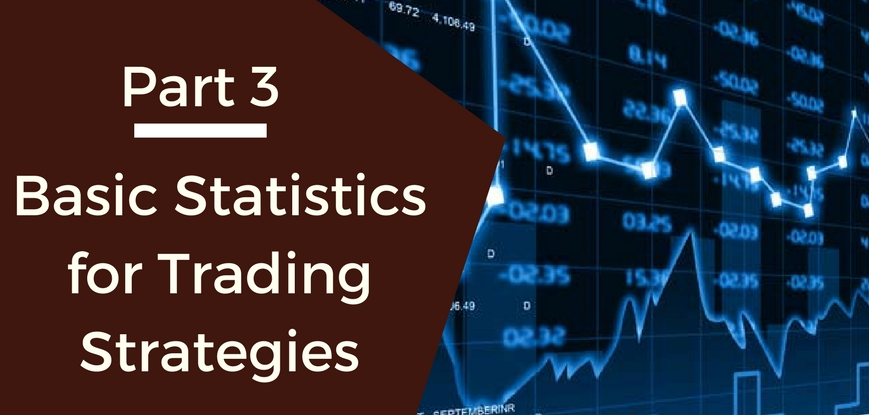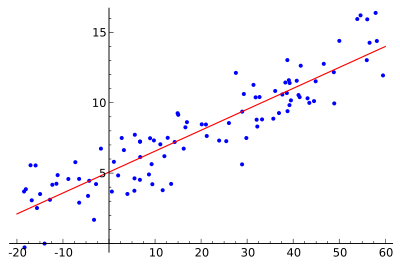# Basic Statistics for Trading Strategies (Part 3) - Regression, Correlation and Co-IntegrationThis post is a part of our series on using statistics and data analysis for trading. In our first post, we discussed summary statistics such as mean, standard deviation, volatility & Bollinger bands. In the second post, we talked about probability distribution functions and logarithmic returns on stock prices.

In this post, we will try to understand the relationship between a stock and a market index. The terms we will understand are regression, correlation and co-integration. This post also tries to answer the basic question in portfolio management: “what is the beta of a stock?”

We will continue working with the dataset used in the previous post: MARUTI SUZUKI India Limited- Daily data from Jan 01, 2013 to Dec 31, 2013. In addition to this, we will use Nifty data for the same time period. You can download the CNX Nifty aggregate price data from the source below:

http://nseindia.com/products/content/equities/indices/historicalindexdata.htm

### CNX Nifty

The CNX Nifty is a well diversified 50 stock index accounting for 23 sectors of the economy. It is used for a variety of purposes such as benchmarking fund portfolios, index based derivatives and index funds.

Our stock, Maruti, is one of the CNX Nifty stocks.

### CNX Nifty and Maruti

Given Maruti is one of the Nifty stocks, the change in Nifty index & Maruti prices should be correlated, that is, change in one should be related to the change in other. Let us find out!

After merging the two data sets by the common column of “Date”, the correlation that we get is 0.55! As expected, the two data sets are positively correlated.

> cor(mergedb\$nifty.returns, mergedb\$maruti.returns)

 0.55

### Understanding correlation

Correlation is a unit free number lying between -1 and 1 which gives us the measurement of the relationship between variables. A highly positive correlation value lying between 0.7 and 1.0 tells us that the change in one variable is positively related to the change in the other variable. That means, if one variable increases, there is a high probability that other one will increase as well. The behavior will be consistent in other cases of decrease or no change in value as well.

On the other hand, a highly negative correlation value lying between -0.7 to -1.0 tells us that the change in one variable is negatively related to the change in the other variable. That means, if one variable increase, there is a high probability that the other one will decrease.

The low correlation value around -0.2 and 0.2 tells us that there is no strong relationship between the two variables.

A point to note is that correlation doesn’t tell us anything about causality. So for instance, it is possible that instances of lung cancers are correlated with the number of cigarettes smoked in a lifetime among a population, that does not establish a causality of smoking to lung cancer. One would be required to do a controlled group study keeping constant all other influential factors to establish such a causality relation. Machine learning based trading models are very good at extracting such causality between different indicactors.

Correlation is the measure of linear relationship. For instance, the correlation between x and x2 might be as close as 0. Even though there is a strong relationship between the two variables, it would not be captured in the correlation value.

Now that we have statistically established that Nifty and Maruti are positively correlated, we would like to do more. We would like to see if, given the Nifty index value, we can predict Maruti prices. A popular measure of volatility or systematic risk for a stock, when compared to the market index, is “beta coefficient”, which is used in the Capital Asset Pricing Model (CAPM) for portfolio management. This model calculates the expected returns of a stock based on the beta and expected market returns.

Beta is calculated using regression analysis.

### Linear Regression

It is a simple technique to model or predict the dependent variable (y) using independent variables (x1, x2, etc). In simple linear regression, there is only one independent variable, x, and one dependent variable, y. The values of x & y are plotted in a scatter-plot such as shown below and a line is drawn which best fits this data, or minimizes the distance from the points to the line.Ref: http://en.wikipedia.org/wiki/Linear_regression

Since our goal is a prediction, we first use the sample data to create a regression model and then use the fitted model for further predictions.

In case of Nifty & Maruti, the linear regression model is

Y =  0.0004 + 0.9349 * X,

where Y represents Log Returns on Nifty Index & X represents Log Returns on Maruti Closing Prices for the same period.

The coefficient of X in the equation above gives the value of beta. Hence, the beta of the stock is 0.9349 in this case. This number is less than 1, representing that the stock’s price will be less volatile than the market. However, it is also very close to 1 and so one can interpret that the stock price maintains the same movement as the market.

R2 = 0.3088 which is a small number, tells us that the variance of Maruti returns and variance of index returns are not strongly related.

The beta value is used by some risk managers to diversify their portfolio so that they have a mix of different beta stocks so as to earn profits as per their risk appetite.

Beta is calculated using the historical data over a period of time without accounting for market trend during that time. Therefore, the beta value does not guarantee the future movement in stock prices.

### Next Step

Are you keen to learn various aspects of Algorithmic trading to enhance your existing skill set or to start trading on your own? Check out the Executive Programme in Algorithmic Trading (EPAT®). The course covers training modules like Statistics & Econometrics, Financial Computing & Technology, and Algorithmic & Quantitative Trading. EPAT® equips you with the required skill sets to be a successful trader. Enroll now to being your career in Algorithmic Trading.